##### Matrix Algebra# Introduction to Econometrics - Matrix Algebra

 What follows under this section, is an introduction to matrix algebra which is essential in order to understand the discussion of more advanced econometrics and statistics. Most properties are only defined or described without giving examples, exercises, or rigorous proofs. In any case remember, mathematics is pure fun! We write a column and row vector as follows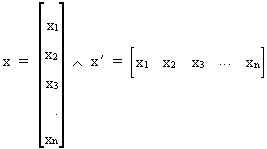(I.IV-1) where the prime (') is an indication of transposition. The length of a vector is defined as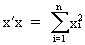(I.IV-2) Note the following, obvious relationships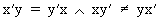(I.IV-3) We write a n*m matrix as follows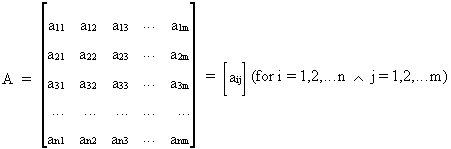(I.IV-4) Addition of matrices can be defined as(I.IV-5) whereas multiplication can be written as(I.IV-6) Transposition of matrices has two simple properties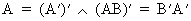(I.IV-7) The multiplication of two diagonal matrices results in a diagonal matrix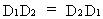(I.IV-8) where a 'diagonal matrix' can be defined as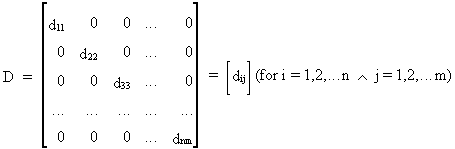(I.IV-9) A special diagonal matrix is a matrix where all diagonal elements are equal to one (identity matrix denoted I). Therefore, it is obvious that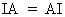(I.IV-10) A matrix with only zero elements is neutral in addition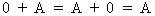(I.IV-11) Vectors are told to be linearly independent if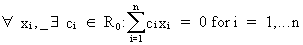(I.IV-12) Now we are able to define the rank of a matrix as the number of linearly independent rows or columns. Also note that all zero matrices have rank = 0. The rank of a (m*n) matrix is equal to the rank of the largest sub matrix with a determinant different from zero where the determinant of a matrix is defined by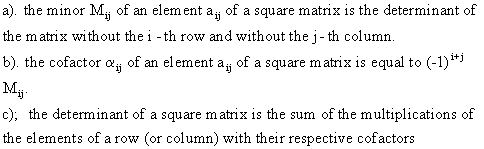Note that a determinant of a two by two matrix can be found by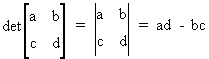(I.IV-13) It is also quite obvious that the rank of a product of two matrices is less or equal to the rank of either the one or the other matrix. Transposition does not alter the rank of a matrix. If a square matrix has a full rank (rank equal to the number of rows or columns) we call this matrix not singular. Furthermore, if we have a square non singular matrix then the inverse of a matrix A can be defined as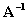if and only if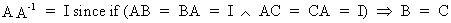(I.IV-14) The inverse of an inverse matrix, is equal to the original matrix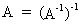(I.IV-15) and the inverse of the transposed is the transposition of the inverse matrix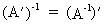(I.IV-16) A diagonal matrix is non singular if, and only if all diagonal elements are different from zero. Above this, the inverse of such a matrix can be found by writing a diagonal matrix where all diagonal elements are replaced by their respective reciprocals. The determinant of a product AB (if AB exists) is equal to the product of the determinants if both matrices are square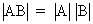(I.IV-17) The rank of AB and the rank of CA are equal to the rank of A if B and C are non singular. If A and B are of the same order then(I.IV-18) Ifthen M is said to be idempotent. In our discussions we'll also always assume that these idempotent matrices are symmetric (which means that the element of the i-th row and the j-th column is equal to the element of the j-th row and the i-th column). An important example of an idempotent matrix is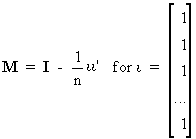(I.IV-19) which can be shown quite easily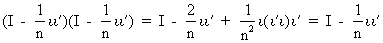(I.IV-20) Idempotent matrices are very important in order to write variables as deviations from the mean. For instance, if B is an observation matrix and M is the idempotent matrix of (I.IV-20), then we can write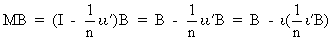(I.IV-21) where obviously all columns of B have been written as their deviation from the mean. Even the sum of the square products B'B of a square matrix B can be written as deviations from the mean: (MB)'MB = B'MB (where M is again idempotent). When using two different matrices B and C it is still possible to write the deviations from the mean of the sum of square products (B'C) as follows: (MB)'MC = B'MC. Define the trace of a square matrix A as(I.IV-22) It is obvious that the following rules hold for the trace of a matrix: a) tr (k A) = k tr A where k is a real number b) tr(A+B) = tr A + tr B c) tr (AB) = tr (BA) d) tr A = rank A if A is idempotent A quadratic form associated with a symmetric square matrix A is defined as the scalar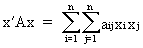(I.IV-23) (A is considered to be a square symmetric matrix). A is called a positive definite matrix if and only if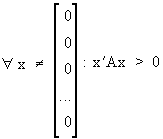(I.IV-24) A positive semi definite matrix is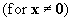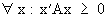(I.IV-25) Obviously a negative definite and a negative semi definite matrix can be defined analogously. An indefinite matrix however is defined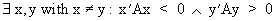(I.IV-26) The matrix A is positive definite if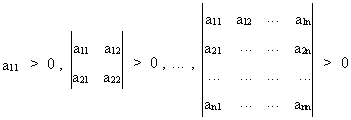(I.IV-27) All principal minors and the determinant of a matrix A are positive if A is positive definite. A very important property is that all positive definite matrices are non singular! If A is positive definite (pos. semi def.) and B is non singular then B'AB is also positive definite (pos. semi def.). If there exists a m*n matrix A with rank mNo news at the moment...© 2000-2018 All rights reserved. All Photographs (jpg files) are the property of Corel Corporation, Microsoft and their licensors. We acquired a non-transferable license to use these pictures in this website.
The free use of the scientific content in this website is granted for non commercial use only. In any case, the source (url) should always be clearly displayed. Under no circumstances are you allowed to reproduce, copy or redistribute the design, layout, or any content of this website (for commercial use) including any materials contained herein without the express written permission.

Information provided on this web site is provided "AS IS" without warranty of any kind, either express or implied, including, without limitation, warranties of merchantability, fitness for a particular purpose, and noninfringement. We use reasonable efforts to include accurate and timely information and periodically updates the information without notice. However, we make no warranties or representations as to the accuracy or completeness of such information, and it assumes no liability or responsibility for errors or omissions in the content of this web site. Your use of this web site is AT YOUR OWN RISK. Under no circumstances and under no legal theory shall we be liable to you or any other person for any direct, indirect, special, incidental, exemplary, or consequential damages arising from your access to, or use of, this web site.

Contributions and Scientific Research: Prof. Dr. E. Borghers, Prof. Dr. P. Wessa
Please, cite this website when used in publications: Xycoon (or Authors), Statistics - Econometrics - Forecasting (Title), Office for Research Development and Education (Publisher), http://www.xycoon.com/ (URL), (access or printout date).